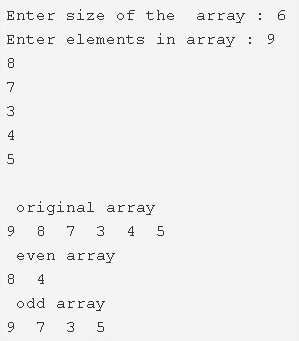# C Program To Put Even And Odd Elements Of Array Into Two Separate Arrays

C Program to put even and odd elements of an array into two separate arrays – In this article, we will discuss the several ways to put even and odd elements of an array into two separate arrays in C programming.

Suitable examples and sample programs have also been added so that you can understand the whole thing very clearly. The compiler has also been added with which you can execute it yourself.

The means discussed here are as follows:

• Using Standard Method
• Using Function

As the name of the title suggests, we will be having both even and odd elements in a given array.As we can see in the photo above, we have a bunch of elements in the given array. Those elements are:

9, 8, 7, 3, 4 and 5.

Out of these, 8 and 4 are even. Rest of the lot is odd in nature.

Thus, the various methods to separate odd and even elements present in an array in C programming is as follows:

## Using Standard Method

1. Read the size of the array and store the value into the variable n.

2) The scanf(“%d”,&a[i]) reads the elements and store the elements into the array a[] using for loop for(i=0;i<n;i++).

3) The main() calls the print() function by passing array,array size as arguments, to print the original array.

4) j=k=0, for loop iterates from i=0 to i<n

a) If the remainder of a[i]/2 is zero then a[i] is an even number and store the even number into the array b[] as b[j++]=a[i].

b) If the remainder of a[i]/2 is not equal to zero then a[i] is an odd number and store the odd number into the array c[] as c[k++]=a[i].

5) After all iterations of for loop,

a) Print the b[] array which is having even numbers, using the function print(b,j).

b) Print the c[] array which is having odd numbers, using the function print(c,k).

Output:

### Using Function

1. The main() calls the function() by passing three arrays a,b,c and array size as arguments.

2) The function() separates the even numbers and odd numbers from the original array as follows,

The for loop iterates from i=0 to i<n with the structure for(i=0;i<n;i++)

a) If the remainder of a[i]/2 is zero then a[i] is even number and store that even number into the array b[] as b[j++]=a[i].

b) If remainder is not equal to zero then a[i] is odd number and store that odd number into the array c[] as c[k++]=a[i].

c) function() prints the even numbers by calling print(b,j) and it prints odd numbers by calling print(c,k).

Output:
x

## C Program To Print Number Of Days In A Month | Java Tutoring

C program to input the month number and print the number of days in that ...